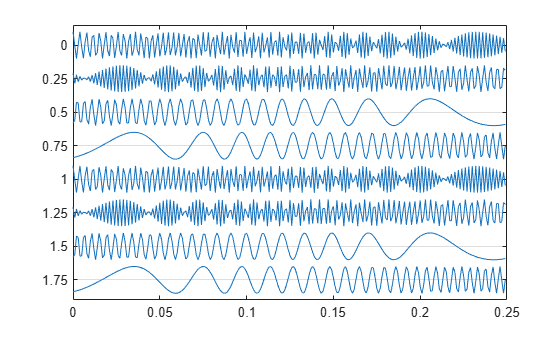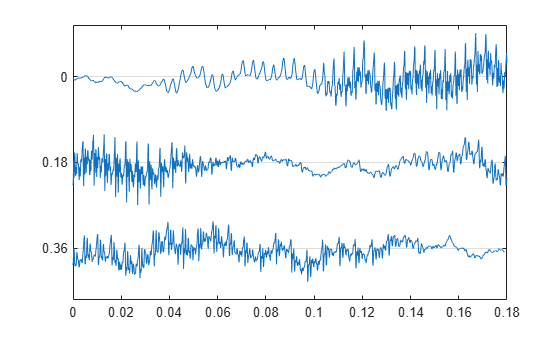# strips

## Syntax

``strips(x)``
``strips(x,n)``
``strips(x,sd,fs)``
``strips(x,sd,fs,scale)``

## Description

````strips(x)` plots `x` in horizontal strips of length 250.```
````strips(x,n)` plots `x` in strips that are each `n` samples long.```

example

````strips(x,sd,fs)` plots `x` in strips of duration `sd` given the sample rate of `fs` samples per second.```

example

````strips(x,sd,fs,scale)` also scales the vertical axes.```

## Examples

collapse all

Plot two seconds of a frequency-modulated sinusoid in 0.25-second strips. Specify a sample rate of 1 kHz.

```fs = 1000; t = 0:1/fs:2; x = vco(sin(2*pi*t),[10 490],fs); strips(x,0.25,fs)```Load a speech signal sampled at ${F}_{s}=7418\phantom{\rule{0.2777777777777778em}{0ex}}Hz$. The file contains the recording of a female voice saying the word "MATLAB®."

`load mtlb`

Plot the signal in 0.18-second long strips. Scale the vertical axes to 125%.

`strips(mtlb,0.18,Fs,1.25)`## Input Arguments

collapse all

Input signal, specified as a vector or matrix. If `x` is a matrix, the `strips` function plots each column of `x` as a horizontal strip on the same plot. The function ignores the imaginary part of complex-valued `x`.

Data Types: `single` | `double`
Complex Number Support: Yes

Length of strips, specified as a real positive scalar.

Duration in seconds, specified as a real positive scalar. If `sd` is specified, then you must also specify `fs`.

Sample rate, specified as a real positive scalar. `fs` has units of hertz.

Scale factor, specified as a scalar. The `strips` function ignores the imaginary part of complex-valued `scale`.

Data Types: `single` | `double`
Complex Number Support: Yes